### Drones

6 results in Drones after filtering by Colour
Model Brand Release year Battery capacity (Wh) Length (cm) Weight (kg) Colour Maximum flying height (m) Maximum working altitude (m) Battery charge (Ah) Takeoff weight (g) Battery weight (g) Charging time (min) Maximum pitch velocity (°/s) Width (cm) Maximum yaw velocity (°/s) Height (cm) Maximum video bitrate Maximum horizontal speed (km/h) Maximum focal length (mm) Depth (cm) Maximum operating temperature (°C) Maximum flight time (min) Maximum tilt angle (°) Flying time (min) Minimum focal length (mm) Folded length (cm) Resolution (MP) Minimum recommended age (y) Voltage (V) Gyro axes Folded height (cm) Folded width (cm) Internal storage (GB) Battery voltage Maximum ascent speed (m/s) Operating frequency (GHz) Transmission frequency (GHz) Maximum descent speed (m/s) Range (km) Minimum aperture (f) Sensor size (") Number of USB A ports Maximum shutter speed Number of USB C ports Maximum range (km) Minimum operating temperature (°C) Camera? GPS? SD card slot? GLONASS? Folding? Retractable landing gear Barometer? Magnetometer? Form factor VR compatible Video format Battery type Angle style Motor Sensor type Flight control system Buy now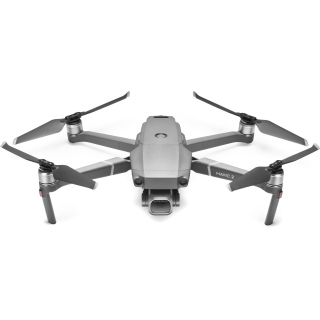Mavic 2 DJI 2018 [x] 28.4cm [x] Black [x] 90min [x] 28cm [x] 11.2cm [x] 31min [x] No [x] MP4 [x] / MOV (MPEG-4 AVC/H.264, HEVC/H.265) [x]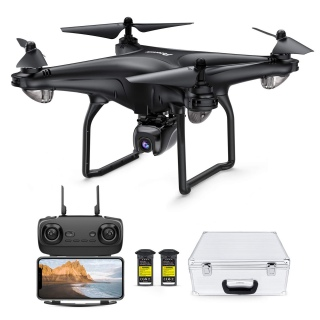D58 Potensic 2018 [x] Black [x] 18min [x] 7.4 [x] Yes [x]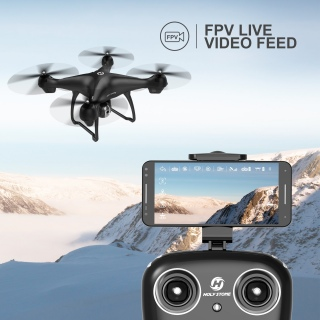HS100 Holy Stone 50cm [x] 0.7kg [x] Black [x] / Red [x] 0.25Ah [x] 700g [x] 180min [x] 50cm [x] 17.5cm [x] 15min [x] 3.7V [x] 2.4GHz [x] 2.4GHz [x] 0.5km [x] Yes [x] No [x] Quadcopter [x] Lithium-polymer [x]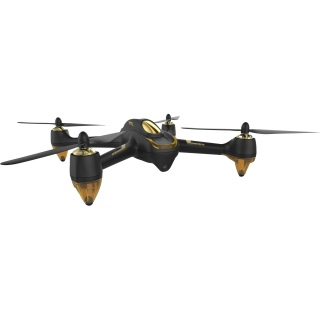H501S Hubsan Black [x] 210min [x] 20min [x]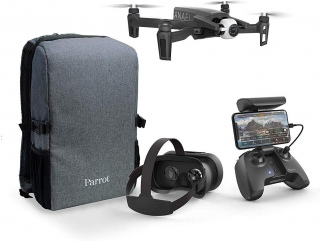Anafi FPV Parrot 24.1cm [x] 0.315kg [x] Black [x] 4500m [x] 2.5Ah [x] 31.5cm [x] 6.4cm [x] 55km/h [x] 40°C [x] 26min [x] 26min [x] 21.8cm [x] 6.4cm [x] 6.9cm [x] 3.6 [x] 4km [x] 1 [x] 1 [x] -10°C [x] Yes [x] Yes [x] Yes [x] Quadcopter [x]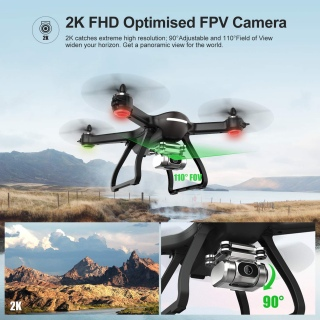HS700D Holy Stone 21.99cm [x] 0.608kg [x] Black [x] 2.8Ah [x] 420min [x] 21.99cm [x] 15.49cm [x] 22min [x] 2.4GHz [x] 1km [x] / 0.39km [x]